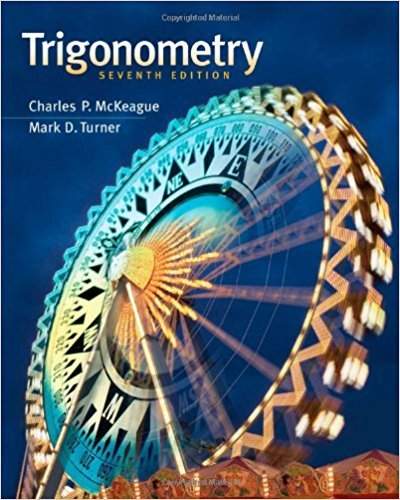×
Log in to StudySoup
Get Full Access to Trigonometry - Textbook Survival Guide
Join StudySoup for FREE
Get Full Access to Trigonometry - Textbook Survival Guide

Already have an account? Login here
×
Reset your password

# Solutions for Chapter 4.3: Vertical and Horizontal Translations## Full solutions for Trigonometry | 7th Edition

ISBN: 9781111826857Solutions for Chapter 4.3: Vertical and Horizontal Translations

Solutions for Chapter 4.3
4 5 0 304 Reviews
20
5
##### ISBN: 9781111826857

This textbook survival guide was created for the textbook: Trigonometry, edition: 7. This expansive textbook survival guide covers the following chapters and their solutions. Chapter 4.3: Vertical and Horizontal Translations includes 88 full step-by-step solutions. Trigonometry was written by and is associated to the ISBN: 9781111826857. Since 88 problems in chapter 4.3: Vertical and Horizontal Translations have been answered, more than 41606 students have viewed full step-by-step solutions from this chapter.

Key Math Terms and definitions covered in this textbook
• Affine transformation

Tv = Av + Vo = linear transformation plus shift.

• Associative Law (AB)C = A(BC).

Parentheses can be removed to leave ABC.

• Change of basis matrix M.

The old basis vectors v j are combinations L mij Wi of the new basis vectors. The coordinates of CI VI + ... + cnvn = dl wI + ... + dn Wn are related by d = M c. (For n = 2 set VI = mll WI +m21 W2, V2 = m12WI +m22w2.)

• Cofactor Cij.

Remove row i and column j; multiply the determinant by (-I)i + j •

• Eigenvalue A and eigenvector x.

Ax = AX with x#-O so det(A - AI) = o.

• Elimination matrix = Elementary matrix Eij.

The identity matrix with an extra -eij in the i, j entry (i #- j). Then Eij A subtracts eij times row j of A from row i.

• Four Fundamental Subspaces C (A), N (A), C (AT), N (AT).

Use AT for complex A.

• Fundamental Theorem.

The nullspace N (A) and row space C (AT) are orthogonal complements in Rn(perpendicular from Ax = 0 with dimensions rand n - r). Applied to AT, the column space C(A) is the orthogonal complement of N(AT) in Rm.

• Gram-Schmidt orthogonalization A = QR.

Independent columns in A, orthonormal columns in Q. Each column q j of Q is a combination of the first j columns of A (and conversely, so R is upper triangular). Convention: diag(R) > o.

• Hessenberg matrix H.

Triangular matrix with one extra nonzero adjacent diagonal.

• Length II x II.

Square root of x T x (Pythagoras in n dimensions).

• Markov matrix M.

All mij > 0 and each column sum is 1. Largest eigenvalue A = 1. If mij > 0, the columns of Mk approach the steady state eigenvector M s = s > O.

• Multiplication Ax

= Xl (column 1) + ... + xn(column n) = combination of columns.

• Pseudoinverse A+ (Moore-Penrose inverse).

The n by m matrix that "inverts" A from column space back to row space, with N(A+) = N(AT). A+ A and AA+ are the projection matrices onto the row space and column space. Rank(A +) = rank(A).

• Random matrix rand(n) or randn(n).

MATLAB creates a matrix with random entries, uniformly distributed on [0 1] for rand and standard normal distribution for randn.

• Spectrum of A = the set of eigenvalues {A I, ... , An}.

Spectral radius = max of IAi I.

• Symmetric matrix A.

The transpose is AT = A, and aU = a ji. A-I is also symmetric.

• Trace of A

= sum of diagonal entries = sum of eigenvalues of A. Tr AB = Tr BA.

• Vandermonde matrix V.

V c = b gives coefficients of p(x) = Co + ... + Cn_IXn- 1 with P(Xi) = bi. Vij = (Xi)j-I and det V = product of (Xk - Xi) for k > i.

• Volume of box.

The rows (or the columns) of A generate a box with volume I det(A) I.